# Logarithms and Age CountingI had just finished reading his book La Science à l’usage des non-scientifiques when, sadly, Albert Jacquard, aged 2.06, passed away, on September 11, 2013.

What? He was 2.06?

That’s how he liked his age to be given! And, to pay tribute to this renowned geneticist and great science popularizer, I’ve decided to write this article to explain how and why he liked ages to be given that way!

## Logarithms and Scales

Before getting to age counting, let me first introduce logarithms, which are the key mathematical objects to describe ages as Albert Jacquart liked to do it.

Logarithms? What the hell is that?

The logarithm is an operator to solve equations like $10^x = 100$.

The solution is 2, right?

Yes! That’s why we write $\log_{10} (100) = 2$.

But what’s the point in solving such an equation?

Mathematicians like to study the solutions of equations… But more practically, the logarithm is an amazing tool to write down in a readable way extremely huge or extremely small number. For instance, the logarithms of all the scales of the universe only range between -35 and 27! This is what’s remarkable with logarithms! They enable to capture all the scales of the complex universe with two-digit numbers! These scales are obtained with logarithms are called logarithmic scales. For an awesome example, check this awesome animation by Cary Huang on htwins.net.

The fact that all scales of our universe lie within 2-digit logarithmic values actually highlights how small astronomical figures are. In comparison, mathematics and cryptography often deal with much larger numbers, whose logarithms can equal millions! Find out more about very big numbers with my talk in A Trek through 20th Century Mathematics.
So, logarithmic scales are great to talk about scales of the universe?

Yes! But that’s not all. In fact, that’s not where they are used the most! Many other measurements are made with such logarithm scales. This is the case, for instance, of decibels, used to measure the intensity of signals, like in acoustics or photography, as you can read it in my article on high dynamic range. In both cases, sensors like our ears, eyes, microphones or cameras have the amazing ability of capturing a very large range of sound or light intensities. For instance, our eyes can see simultaneously a dark spot and a spot a million times brighter, while our ears can hear sounds from 0 to 120 decibels, the latter being 10^12 times louder than the former! Making sense of these large scales is more doable with logarithmic scales!

Another important example is the measure of acid concentration in water (the pH), as explained in this great video by Steve Kelly on TedEducation:

So, if I get it right, logarithms aren’t necessarily in base 10, right?

Yes. But let’s talk about other bases later…

What do you want to talk about?

One area in which logarithms are essential is to describe of growth. Because logarithms capture huge numbers with small numbers, it means that it takes an extremely huge numbers for its logarithm to be big. Mathematically, we say that logarithms have smaller growth than any function $x^\alpha$ for $\alpha > 0$. Thus, we often use them as a benchmark to discuss small growths. A crucial example of that is the fundamental prime number theorem.

The what theorem?

The prime number theorem is a characterization of the distribution of primes. First observed by Carl Friedrich Gauss, it was later proved by Hadamard and de la Vallée-Poussin. It says that the average gap between consecutive primes grows as we consider bigger and bigger numbers, and this growth is the same as the growth of logarithms. But, rather than my explanations, listen to Marcus du Sautoy’s:

What I find particularly puzzling with the prime number theorem is that the logarithm involved is actually the natural logarithm we’ll talk about later! As you’ll see, the definition of the natural logarithm has absolutely nothing to do with primes!

Other important examples of comparisons of growth appear in complexity theory. You can read about it, for instance, in my article on parallelization.

## Age Counting

From a mathematical perspective, being able to write down huge numbers isn’t a ground-breaking achievement. That’s not what makes logarithms essential to mathematics. What does is their ability to transform multiplications into additions. And that’s the key property of logarithms which led Albert Jacquart to propose to use them to define ages.

What do you mean?

Albert Jacquart noticed that there was not as much age difference between a 25-year old girl and a 45-year old man as there is between a 5-year old girl and a 25-year old. This idea is illustrated by the following epic extract from Friends, where Monica tells her parents that she is dating their old friend Richard:

Humm.. That’s funny! Age difference seems to decrease… with age…

Well that’s not that surprising when you think about it. After all, a 25-year old man has lived 5 times longer than a 5-year old girl, but there’s not such a big ratio between a 45-year old man and a 25-year old girl.

So you’re saying that age difference should be counted in terms of age ratio rather than… age difference?

Well, sort of… According to Albert Jacquart, the reason why age difference doesn’t mean what it should mean is because we’re not using the right unit to measure age!

What? Our measure of time is wrong?

Our measure of time is fine. But what’s misleading is our way of saying how old we are! In particular, a right way to express age difference should rather correspond to the ratio of the amounts of time lived.

How do we do that?

The first step is to compare lifetime to a relevant characteristic amount of time which represents life. Albert Jacquart liked to choose the human gestation duration (9 months). Then, he proposed to write the number of gestation duration we have lived as a power of 10. And this power would then be defined as the age. In other words, the age is now defined as the logarithm of the number of gestation durations one has lived. This corresponds to the following formulas:Recall, that the Age in the formula is how Albert Jacquart defines it! It’s not what we usually call age!

So, how old are Monica and Richard?

25-year-old Monica has lived 300 months. That’s $300/9 = 33$ gestation durations. Thus, her age is $\log_{10}(33) \approx 1.52$. Meanwhile, 45-year-old Richard has age $\log_{10}(45 \times 12/9) \approx 1.78$.

This all sounds complicated… Why should we do that?

Well, we said that what matters is the ratio of the amount of times people have been alive, right? So, to compare the ages of Richard and Monica, we would have to divide the respective Richard’s lifetime by Monica’s lifetime… And, magically, we obtain the following equation:So the ratio of lifetimes now corresponds to… an actual age difference! That’s what we wanted!

So what are the age differences between Monica and Richard at the time of the episode and 20 years earlier?

The age difference between 25-year-old Monica and 45-year-old Richard is $1.78-1.52 \approx 0.26$. Twenty years before that, the age difference was $\log_{10}(25 \times 12/9) – \log_{10}(5 \times 12/9) = 0.70$. Compared to twenty years earlier, Monica and Richard are now nearly the same age! Plus, wait another 20 years, and their age difference would then be 0.16…

I know most people don’t like decimal numbers. To fix this, we can choose another base for the logarithm, or, more simply, multiply all ages by 100! And before you go around and say this is nuts, it’s actually the core of Weber-Fechner law!

## Products Become Sums

This ability logarithms have to transform multiplication into addition is the core of its potency in mathematics. Before computers were invented, this yielded a powerful way to quickly compute huge multiplications, as explained in the following video by Numberphile:

But with the invention of computers, this has become completely useless, right?

Pretty much! But that’s not the only application of the ability of logarithms to transform products into sums. In statistics, to adjust models, one classical technics consists in searching for parameters which make the observations the most likely. This is known as the maximum likelihood estimation method. It’s the one I used to estimate the levels of national football teams to simulate world cups!

What do logarithms have to do with that?

The likelihood is then a probability of a great number of events occurring. Assuming these events independent, the likelihood then equals the multiplication of the probabilities of the events. Yet, to maximize the likelihood, the classical approach consists in differentiating it. And, as you’ve probably learned it, differentiating a product is quite hard. The awesomeness of logarithms is to transform the product into a sum, which is infinitely easier to differentiate!

The moral is that, whenever you must differentiate a complex product, try to differentiate its logarithm instead!

I’ll keep that in mind!

Another area where logarithms are essential is Shannon’s information theory and entropy in thermodynamics. In particular, to express the amount of information a system can contain, Shannon had the brilliant idea to consider it to be the logarithm of the number of states it can be in.

Why is that such a great idea?

When you have two hard drives, the number of states they can be in is the product of the number of states each can be in. By using Shannon’s quantification of information, called entropy, the amount of information two hard drives can contain is now the sum of the amounts of information of each of them! That’s what we really mean when we say that 1 Gigabytes plus 1 Gigabytes equals 2 Gigabytes! Behind this simple sentence lies the omnipotence of logarithms!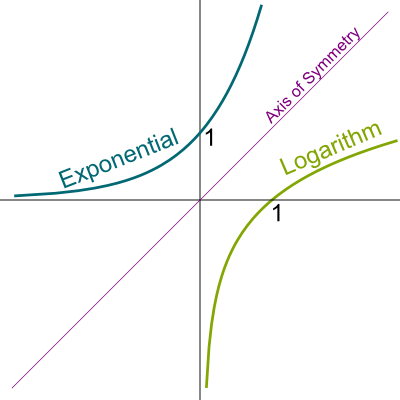From a pure mathematical viewpoint, this ability of logarithms to transform products into additions is a fundamental connection between the two operations. We say that logarithms induce an equivalence between products of positive numbers and sums of real numbers.

More precisely, the set of logarithms is exactly the set of continuous group isomorphisms from $(\mathbb R^*_+, \times)$ to $(\mathbb R, +)$. In fact, the logarithm is even differentiable, and its inverse is too, making the logarithm a Lie group diffeomorphism!
Does that mean that we can go the other way around?

Yes! The other ways around are known as exponentials. Exponentials transform sums into products. Just like logarithms, exponentials are defined by a base. If an exponential and a logarithm are defined with the same base, then the exponential of the logarithm and the logarithm of the exponential get us back to our initial point. For instance, $\log_{10} (10^x) = 10^{\log_{10}(x)} = x$. Geometrically, this beautiful property means that the main diagonal is an axis of symmetry between the graphs of exponentials and the graphs of logarithms, as displayed in the figure on the right.

## Calculus and Natural Logarithm

The area where logarithms have strived the most is calculus, especially in differential and integral calculus.

Why?

That’s because the primitive of $1/x$ is… a logarithm!

If you don’t know what a primitive is, don’t be scared! I’ll explain everything!
A logarithm? Why on earth would that be?

Hehe!!! Let’s prove it! Let’s show that the primitive of $1/x$ transforms multiplications into additions. Since only logarithms can do that continuously, this will prove that the primitive must be a logarithm.

Wait… What’s a primitive?

A primitive is a measure of the area below the curve. In our case, the primitive we will be focusing on equals the area below the curve $1/x$ between $1$ and $X$, as described below. Let’s call it $Area(1,X)$, instead of its usual complicated notation $\int_1^X dx/x$.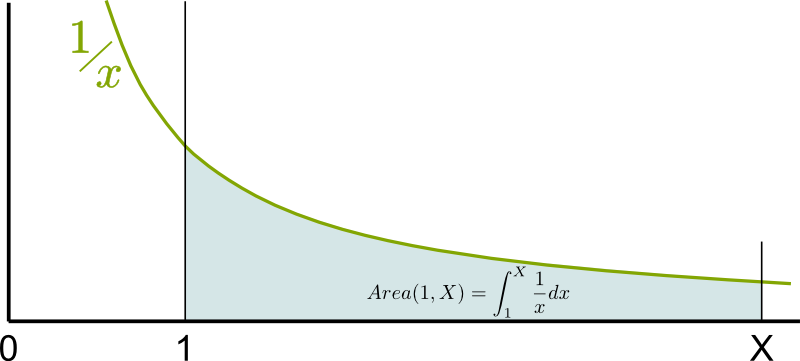Note that if $X$ is actually in the left of 1, then $Area(1,X)$ is the opposite of the area under the curve between $X$ in $1$. In other words, $Area(1,X) = – Area(X,1)$.

Now, I want you to prove that $Area(1,X)$ is actually a logarithm of $X$!

What? I thought I was only supposed to read!

Come on! It’s a cool exercise!

I have no idea where to start!

Read what I’ve just said earlier!

You said something about proving that the primitive transforms multiplications into additions…

Yes! What does that mean?

I guess it means that I have to prove that $Area(1,X \times Y) = Area(1,X) + Area(1,Y)$…

Exactly!

But how on earth can I prove that?

When I’m stuck, I like to doodle…

Good idea! Let me draw the three areas!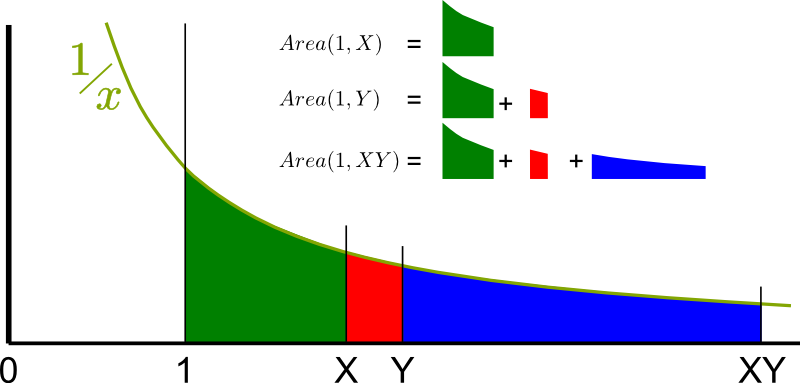So, to prove $Area(1,X \times Y) = Area(1,X) + Area(1,Y)$, what you really need to prove is that…

The green area is the same as the blue one!

Exactly! Technically, what you’ve just used is Chasles relation $Area(1, X \times Y) = Area(1, Y) + Area(Y, XY)$. By then subtracting $Area(1,Y)$ in both sides of the equation above, the equation to prove then becomes $Area(Y, XY) = Area(1,X)$. That’s the equality of the green and blue areas!

Awesome!

But you’re not done yet…

I know… But how can I prove that the green and blue areas are equal?

Compare them!

Humm… I know! For one thing, the blue area is a horizontal stretching of the green one by a factor $Y$!

Exactly! The blue area is horizontally $Y$ times longer! What about vertically?

I know! If we then contract the green area vertically by a factor $Y$, its area won’t have changed!

Bingo! Here’s a figure of the operations you are talking about!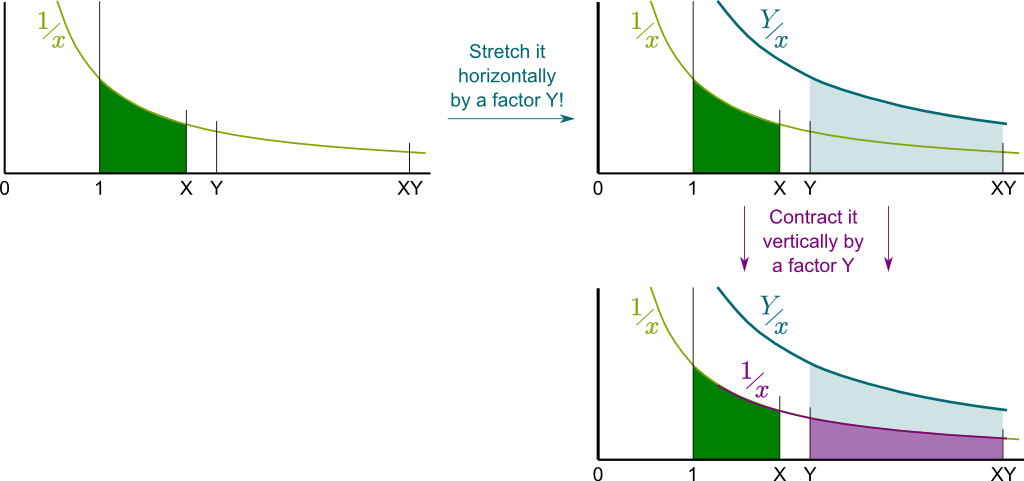To be fair, you’d still need to prove that the horizontally stretched and vertically contracted green area perfectly matches the original blue area. But I’ll leave that as an homework exercise!
That’s why the green and blue areas area equal… And $Area(1, XY) = Area(1,X) + Area(1,Y)$! That’s brilliant!

I know! That’s why the primitive of $1/x$ is a logarithm! It’s known as the natural logarithm, and is commonly denoted $\ln x = Area(1,x)$. The base of this logarithm is a weird number though, called Euler’s number in reference to the great mathematician Leonhard Euler. It is commonly denoted $e$ and is approximately $e \approx 2.7$. It stands for the solution to the equation $Area(1,x) = 1$.

But how does $\ln$ compare to $\log_{10}$?

You should try to figure it out yourself!

Come on! I’ve just proved a difficult theorem!

To find out how to change base, you can simply play around with formulas. Eventually, you’ll obtain $\log_c x = \log_b x / \log_b c$. Thus, in particular, $\ln x = \log_{10} x / \log_{10} e$.

## Power Series

This last section is going to be more technical… A read of my article on differential calculus and infinite series is advised. If you’re not familiar with these important topics of mathematics, you should still be able to follow the main ideas though.

Historically, the invention of logarithms was accompanied with the first studies of infinite sums, also known as infinite series. In particular, power series were to provide deep insights into common diverse functions, including logarithms.

What’s a power series?

A power series is an infinite sum of terms $a_n$ multiplied by $x^n$. We write it $\sum a_n x^n$, and it sort of means $a_0 + a_1 x + a_2 x^2 + a_3 x^3 + \ldots$, and so on to infinity. But, as you can read it in my article on infinite series where I explain why $1+2+4+8+16+… = -1$, infinite sums can be tricky!

What do they have to do with logarithm?

Amusingly, any of the usual functions we use can be written as a power series. The function is then uniquely identified by the terms $a_n$ of the series of $\sum a_n x^n$. And this is the case of logarithms! Sort of…

What do you mean?

Well, actually, the function we should try to write as series is rather $\ln(1+x)$… So, without further ado, let’s find the terms $a_n$ corresponding to $\ln(1+x)$!

How can we do that?

First, we write $\ln(1+x) = \sum a_n x^n$. Then, we’ll use the differential properties of the natural logarithm we have found out earlier.

Are you referring to the fact that the natural logarithm is the primitive of $1/x$?

Yes! This means that if we differentiate the natural logarithm, we should obtain is $1/x$. Now, if you remember your courses of computation of derivatives, you should now be able to compute the derivative of $\ln(1+x)$!

If you’ve forgotten, note that the derivative of $f(g(x))$ is $g'(x) f'(g(x))$…
I’ve found $1/(1+x)$!

Excellent! Now, let’s find the power series of $1/(1+x)$! The key is for you to remember (or learn about) how to calculate sums of geometric series…

I don’t remember it!

Let me redo the calculation then… We have $\sum x^n = 1 + x^1 + x^2 + x^3 + \ldots = 1 + x(1+x+x^2+ \ldots) = 1 + x \sum x^n$. Therefore, $(1-x) \sum x^n = 1$, and $\sum x^n = 1/(1-x)$.

I remember now! But that’s not $1/(1+x)$…

To get from $1/(1-x)$ to $1/(1+x)$, we just need to replace $x$ by $-x$! This gives us $1/(1+x) = 1/(1-(-x)) = \sum (-x)^n = \sum (-1)^n x^n$. That’s a power series!

But how can we now retrieve the power series of $\ln(1+x)$?

We have $\ln(1+x) = \sum a_n x^n$. If we differentiate both sides, we now have $1/(1+x) = \sum n a_n x^{n-1}$. Arranging both sides then yields $\sum (-1)^n x^n = \sum (n+1) a_{n+1} x^n$. Thus…

$a_{n+1} = (-1)^n/(n+1)$, right?

Yes, or, by replacing $n+1$ by $n$, we have $a_n = (-1)^{n+1}/n$.

Each term $a_n$ could have also been computed by differentiating $\ln(1+x)$ $n$ times, taking the values of the derivatives for $x=0$ and dividing that by $n!$. Such a technic yields the Taylor and Maclaurin series.

We can now write $\ln(1+x)$ as a power series!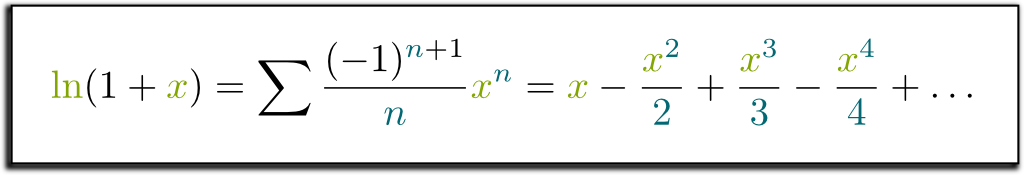Well, sort of…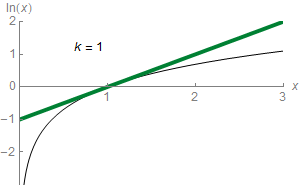What do you mean? Is it not right?

Sadly, you won’t be able to compute $\ln 3$ with this formula, as the power series does not converge for $x=2$! It just gets bigger towards plus infinity and minus infinity alternatively! In fact, as displayed in the animation on the right where terms in the power series are added sequentially to get closer to the actual value of $\sum a_n x^n$, the equality will only hold for logarithms of values between 0 and 2! That’s the horribly everlasting trouble of power series!

Technically, power series always have a convergence radius $R$, possibly infinite. This means that the equality of a power series with the function it stands for will only yield for $-R < x < R$. In our case, the convergence radius equals 1, which means that, for $-1 < x < 1$, we have $\ln(1+x) = \sum (-1)^{n+1} x^n/n$. But this no longer holds if $|x| > 1$.

## Complex Calculus

This last section is going to be even more technical. A read of my article on complex numbers is greatly advised here.

Still, an amazing empowering of the expansion of $\ln(1+x)$ in infinite series is the possibility we now have to define logarithms of complex numbers! Indeed, for any complex number $z$ whose module is smaller than 1, the series $\sum (-1)^{n+1} z^n/n$ converges, and defines a value for $\ln(1+z)$.

In fact, this formula can be used for any algebraic ring. For instance, you can use it to define $\ln(I_n+M)$ when $M$ is a linear endomorphism or a square matrix! The awesome thing is that, providing power series are well-defined, if $MN = NM$, we would always have $\ln(MN) = \ln M + \ln N$, as this property is encoded in the power series expansion!
OK… but this limits us to merely a small area of the complex plane…

Indeed, the values $1+z$ for $|z| <1$ is a disk centered on $1$ and of radius 1. But, amazingly, we can then write the power series of $\ln(c+z)$, for any $c$ such that $\ln c$ has been defined! In another disk now centered on $c$, this will define new values for the complex logarithm $\ln z$! By doing so, we will have expanded the domain of definition of the natural logarithm in the complex plane.

The power series expansion of $\ln(c+z)$ can be deduced, for instance, with the equality $\ln(c+z) = \ln (c(1+z/c)) = \ln c + \ln (1+z/c) = \ln c + \sum (-1)^{n+1} z^n/(nc^n)$. This proves that the radius of convergence is then $|c|$, which means that the origin is at the edge of the disk of convergence.

An example of three first steps of expansions is pictured below: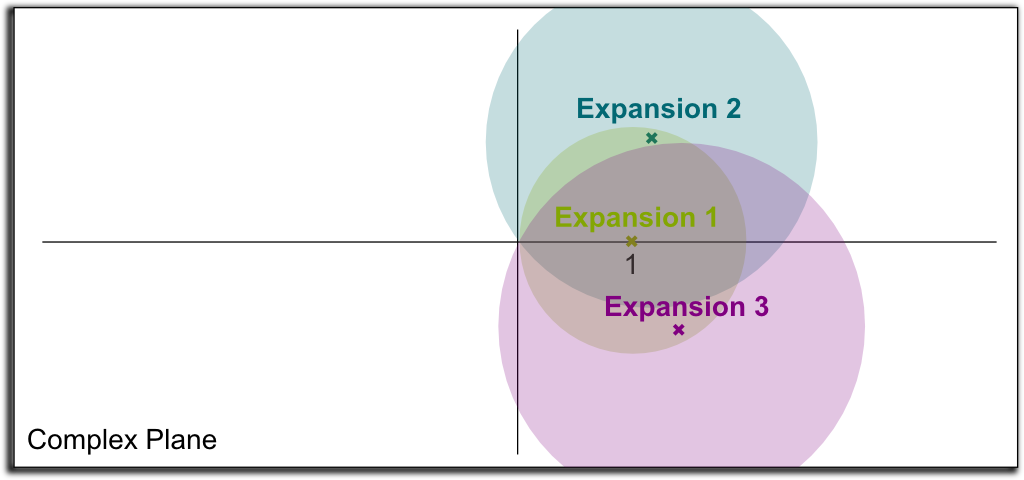By continuing this on to infinity, we can now define the logarithms for nearly all points in the complex plane! This amazing technic is known as analytic continuation.

This idea of analytic continuation is a critical step in Riemann hypothesis, one of the Millenium prize problems and the greatest open problem in number theory.
Will we reach all points in the complex plane?

Some points will be unreachable no matter how hard we try to expand the analytic continuation. But in the case of the logarithm, the only unreachable point is $0$! We say that $0$ is a pole of the natural logarithm.

Can’t we have some contradictory values for the logarithm between two expansions?

Unfortunately, yes we can… The thing is that each expansion is valid locally. Each expansion is in agreement with the expansions of its neighbors. However, as we turn around the origin, we have some expansions which have been built from a clockwise expansion of the original expansion around the origin, while others have been built anti-clockwise. These two kinds of expansions won’t agree. This fundamental result says that the natural logarithm cannot be uniquely expanded to the whole complex plane!

Does it have to do with $e^{2ik\pi} = 1$ for all integers $k$?

Exactly! The natural logarithm is actually defined up to $2i\pi$! That’s why Bernhard Riemann had the brilliant idea of defining the natural logarithm on a sort of infinite helicoidal staircase rather than on a complex plane. This staircase is known as the Riemann surface of the natural logarithm, and is explained by Jason Ross in the video extract below:

To provide a nearly natural well-defined natural logarithm in the complex plane, mathematicians often choose to cut it along a forbidden half line starting at the origin. Typically, the half line of negative number is chosen, and we choose the determination of the logarithm which yields $\ln 1 = 0$. Then, we apply the analytic continuation, but we forbid an analytic continuation to cross the forbidden half line. These restrictions ensure that the expansion of the natural logarithm to the complex plane minus the forbidden half line is well-defined and unique. This is what’s pictured below: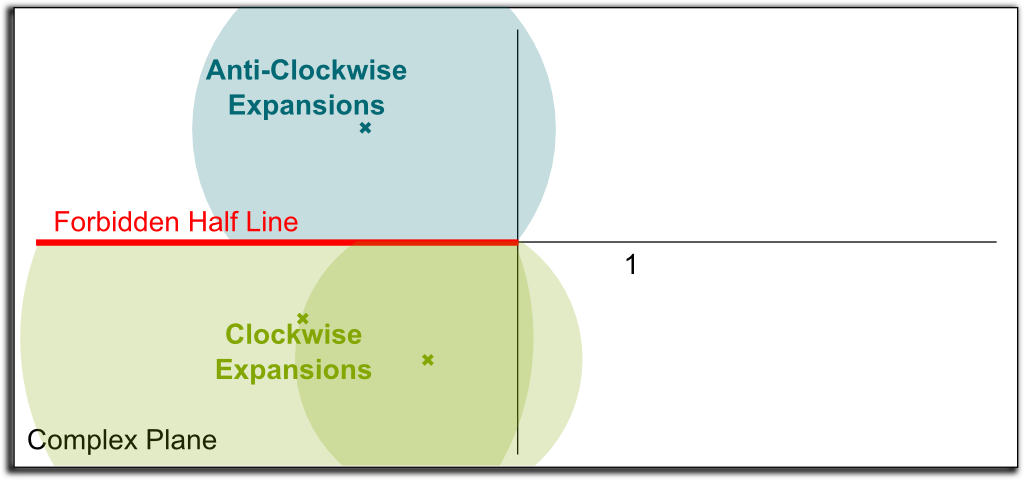The natural logarithm we obtain by doing so is such that $\ln z$ always has an imaginary part in $]-\pi, \pi[$. It is known as the principal value of the logarithm.

## Let’s Conclude

The take-away message of this article is that logarithms are a hidden structure between multiplications and additions. This is the fundamental property of logarithms, and it has many direct applications in computations, calculus and information theory. And age counting… An important implication of that property is the fact that logarithms can capture the size of huge numbers by small ones. This has plenty of applications to measurements in physics and chemistry. It is also essential to describe growths, like in the prime number theorem.

Finally, since we have defined logarithms for complex numbers, let’s mention what happens if we try to define logarithms for other sorts of numbers. In particular, in modular arithmetic, the logarithm modulo $p$ base $b$ of $n$ is naturally defined as the power $x$ such that $b^x$ is congruent to a certain number $n$ modulo $p$. If $p$ is prime, then this logarithm is well-defined. However, computing $\log_{b,p}(n)$ is considered as a difficult problem, and is thus an interesting property for cryptography. This is what’s explained in this great video by ArtOfTheProblem: